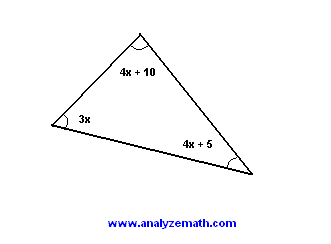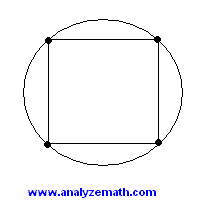# Free GMAT Practice Geometry Problems with Solutions Sample 2

A set of 10 geometry questions similar to the GMAT problem solving, with solutions and expalanations , are presented.

## Question 1

What is the measure of angle x if L1 and L2 are parallel lines?.

A) 130�
B) 50�
C) 60�
D) 90�
E) 180�

## Question 2

Find x..

A) 0
B) 5
C) 10
D) 15
E) 20

## Question 3

ABC is a triangle where AN is perpendicular to CB and BM perpendicular to AC. The length of BC is 10, that of AC is 12 and that of AN is 8. Find the length of BM..

A)
B)
C)
D)
E)

## Question 4

The lengths of sides BA and BC of triangle ABC are equal. Find the measure of angle x..

A)
B)
C)
D)
E)

## Question 5

Find the area of a right isosceles triangle with hypotenuse equal to 24.
A)
B)
C)
D)
E)

## Question 6

The total area of the shape below is equal to 240. Find the length of segment AC..

A)
B)
C)
D)
E)

## Question 7

The circle in the figure has all its vertices on the circle. The area of the square is 144. What is the area of the circle?.

A)
B)
C)
D)
E)

## Question 8

The measures, in degrees, of two consecutive interior angles M and N of a parallelogram are given by 2x + 10 and x + 20 respectively. Find the measures of the two angles.
A)
B)
C)
D)
E)

## Question 9

The perimeter of a rectangle is 90 and its width is 10. What is its area?
A)
B)
C)
D)
E)

## Question 10

What is the ratio of the area of a square of side x to the area of a rectangle of width 2x and length 3x?
A)
B)
C)
D)
E)

## More References and Links to Maths Practice Tests

Free Practice for GAMT Maths tests
Free Compass Maths tests Practice
Free Practice for SAT, ACT Maths tests
Free GRE Quantitative for Practice
Free AP Calculus Questions (AB and BC) with Answers